PyTorch vs TensorFlow: Which Framework Is Best?If you are reading this you've probably already started your journey into deep learning. If you are new to this field, in simple terms deep learning is an add-on to develop human-like computers to solve real-world problems with its special brain-like architectures called artificial neural networks. To help develop these architectures, tech giants like Google, Facebook and Uber have released various frameworks for the Python deep learning environment, making it easier for to learn, build and train diversified neural networks. In this article, we’ll take a look at two popular frameworks and compare them: PyTorch vs. TensorFlow. be comparing, in brief, the most used and relied Python frameworks TensorFlow and PyTorch.

TensorFlow is open source deep learning framework created by developers at Google and released in 2015. The official research is published in the paper “TensorFlow: Large-Scale Machine Learning on Heterogeneous Distributed Systems.”

TensorFlow is now widely used by companies, startups, and business firms to automate things and develop new systems. It draws its reputation from its distributed training support, scalable production and deployment options, and support for various devices like Android.

PyTorch is one of the latest deep learning frameworks and was developed by the team at Facebook and open sourced on GitHub in 2017. You can read more about its development in the research paper "Automatic Differentiation in PyTorch."

PyTorch is gaining popularity for its simplicity, ease of use, dynamic computational graph and efficient memory usage, which we'll discuss in more detail later.

WHAT CAN WE BUILD WITH TENSORFLOW AND PYTORCH?

Initially, neural networks were used to solve simple classification problems like handwritten digit recognition or identifying a car’s registration number using cameras. But thanks to the latest frameworks and NVIDIA’s high computational graphics processing units (GPU’s), we can train neural networks on terra bytes of data and solve far more complex problems. A few notable achievements include reaching state of the art performance on the IMAGENET dataset using convolutional neural networks implemented in both TensorFlow and PyTorch. The trained model can be used in different applications, such as object detection, image semantic segmentation and more.

Although the architecture of a neural network can be implemented on any of these frameworks, the result will not be the same. The training process has a lot of parameters that are framework dependent. For example, if you are training a dataset on PyTorch you can enhance the training process using GPU’s as they run on CUDA (a C++ backend). In TensorFlow you can access GPU’s but it uses its own inbuilt GPU acceleration, so the time to train these models will always vary based on the framework you choose.

TOP TENSORFLOW PROJECTS

Magenta: An open source research project exploring the role of machine learning as a tool in the creative process. (<u>https://magenta.tensorflow.org/</u>

Sonnet: Sonnet is a library built on top of TensorFlow for building complex neural networks. (<u>https://sonnet.dev/</u>)

Ludwig: Ludwig is a toolbox to train and test deep learning models without the need to write code. (<u>https://uber.github.io/ludwig/</u>)

TOP PYTORCH PROJECTS

CheXNet: Radiologist-level pneumonia detection on chest X-rays with deep learning. (<u>https://stanfordmlgroup.github.io/projects/chexnet/</u>)

PYRO: Pyro is a universal probabilistic programming language (PPL) written in Python and supported by <u>PyTorch</u> on the backend. (<u>https://pyro.ai/</u>

Horizon: A platform for applied reinforcement learning (Applied RL) (<u>https://horizonrl.com</u>)

These are a few frameworks and projects that are built on top of TensorFlow and PyTorch. You can find more on Github and the official websites of TF and PyTorch.

COMPARING PYTORCH AND TENSORFLOW

The key difference between PyTorch and TensorFlow is the way they execute code. Both frameworks work on the fundamental datatype tensor. You can imagine a tensor as a multi-dimensional array shown in the below picture.1. MECHANISM: DYNAMIC VS STATIC GRAPH DEFINITION

TensorFlow is a framework composed of two core building blocks:

1. A library for defining computational graphs and runtime for executing such graphs on a variety of different hardware.
2. A computational graph which has many advantages (but more on that in just a moment).

A computational graph is an abstract way of describing computations as a directed graph. A graph is a data structure consisting of nodes (vertices) and edges. It’s a set of vertices connected pairwise by directed edges.

When you run code in TensorFlow, the computation graphs are defined statically. All communication with the outer world is performed via tf.Sessionobject and tf.Placeholder, which are tensors that will be substituted by external data at runtime. For example, consider the following code snippet.This is how a computational graph is generated in a static way before the code is run in TensorFlow. The core advantage of having a computational graph is allowing parallelism or dependency driving scheduling which makes training faster and more efficient.Similar to TensorFlow, PyTorch has two core building blocks:

• Imperative and dynamic building of computational graphs.
• Autograds: Performs automatic differentiation of the dynamic graphs.

As you can see in the animation below, the graphs change and execute nodes as you go with no special session interfaces or placeholders. Overall, the framework is more tightly integrated with the Python language and feels more native most of the time. Hence, PyTorch is more of a pythonic framework and TensorFlow feels like a completely new language.These differ a lot in the software fields based on the framework you use. TensorFlow provides a way of implementing dynamic graph using a library called TensorFlow Fold, but PyTorch has it inbuilt.

2. DISTRIBUTED TRAINING

One main feature that distinguishes PyTorch from TensorFlow is data parallelism. PyTorch optimizes performance by taking advantage of native support for asynchronous execution from Python. In TensorFlow, you'll have to manually code and fine tune every operation to be run on a specific device to allow distributed training. However, you can replicate everything in TensorFlow from PyTorch but you need to put in more effort. Below is the code snippet explaining how simple it is to implement distributed training for a model in PyTorch.3. VISUALIZATION

When it comes to visualization of the training process, TensorFlow takes the lead. Visualization helps the developer track the training process and debug in a more convenient way. TenforFlow’s visualization library is called TensorBoard. PyTorch developers use Visdom, however, the features provided by Visdom are very minimalistic and limited, so TensorBoard scores a point in visualizing the training process.

Features of TensorBoard

• Tracking and visualizing metrics such as loss and accuracy.
• Visualizing the computational graph (ops and layers).
• Viewing histograms of weights, biases or other tensors as they change over time.
• Displaying images, text and audio data.
• Profiling TensorFlow programs.Visualizing training in TensorBoard.

Features of Visdom

• Handling callbacks.
• Plotting graphs and details.
• Managing environments.Visualizing training in Visdom.

4. PRODUCTION DEPLOYMENT

When it comes to deploying trained models to production, TensorFlow is the clear winner. We can directly deploy models in TensorFlow using TensorFlow serving which is a framework that uses <u>REST Client API.</u>

In PyTorch, these production deployments became easier to handle than in it’s latest 1.0 stable version, but it doesn't provide any framework to deploy models directly on to the web. You'll have to use either Flask or Django as the backend server. So, TensorFlow serving may be a better option if performance is a concern.

5. DEFINING A SIMPLE NEURAL NETWORK IN PYTORCH AND TENSORFLOW

Let's compare how we declare the neural network in PyTorch and TensorFlow.

In PyTorch, your neural network will be a class and using torch.nn package we import the necessary layers that are needed to build your architecture. All the layers are first declared in the __init__() method, and then in the forward()method we define how input x is traversed to all the layers in the network. Lastly, we declare a variable model and assign it to the defined architecture (model = NeuralNet()).Recently Keras, a neural network framework which uses TensorFlow as the backend was merged into TF Repository. From then on the syntax of declaring layers in TensorFlow was similar to the syntax of Keras. First, we declare the variable and assign it to the type of architecture we will be declaring, in this case a “Sequential()” architecture. Next, we directly add layers in a sequential manner using model.add() method. The type of layer can be imported from tf.layers as shown in the code snippet below.PROS AND CONS OF PYTORCH AND TENSORFLOW

TENSORFLOW PROS:

• Simple built-in high-level API.
• Visualizing training with Tensorboard.
• Production-ready thanks to TensorFlow serving.
• Easy mobile support.
• Open source.
• Good documentation and community support.

TENSORFLOW CONS:

• Static graph.
• Debugging method.
• Hard to make quick changes.

PYTORCH PROS:

• Python-like coding.
• Dynamic graph.
• Easy & quick editing.
• Good documentation and community support.
• Open source.
• Plenty of projects out there using PyTorch.

PYTORCH CONS:

• Third-party needed for visualization.
• API server needed for production.

PYTORCH AND TF INSTALLATION, VERSIONS, UPDATES

Recently PyTorch and TensorFlow released new versions, PyTorch 1.0 (the first stable version) and TensorFlow 2.0 (running on beta). Both these versions have major updates and new features that make the training process more efficient, smooth and powerful.

To install the latest version of these frameworks on your machine you can either build from source or install from pip

PYTORCH INSTALLATION

●  macOS and Linux

pip3 install torch torchvision

●  Windows

TENSORFLOW INSTALLATION

●  macOS, Linux, and Windows

# Current stable release for CPU-only

pip install tensorflow

# Install TensorFlow 2.0 Beta

pip install tensorflow==2.0.0-beta1

To check if you’re installation was successful, go to your command prompt or terminal and follow the below steps.TENSORFLOW VS PYTORCH: MY RECOMMENDATION

TensorFlow is a very powerful and mature deep learning library with strong visualization capabilities and several options to use for high-level model development. It has production-ready deployment options and support for mobile platforms. PyTorch, on the other hand, is still a young framework with stronger community movement and it's more Python friendly.

What I would recommend is if you want to make things faster and build AI-related products, TensorFlow is a good choice. PyTorch is mostly recommended for research-oriented developers as it supports fast and dynamic training.

Python Face Recognition TutorialIn this video we will be using the Python Face Recognition library to do a few things

Machine Learning In Node.js With TensorFlow.js<p class="ql-align-center">Originally published by James Thomas at dev.to</p><p>Pre-trained models mean developers can now easily perform complex tasks like visual recognitiongenerating music or detecting human poses with just a few lines of JavaScript.</p><p>Having started as a front-end library for web browsers, recent updates added experimental support for Node.js. This allows TensorFlow.js to be used in backend JavaScript applications without having to use Python.</p><p>Reading about the library, I wanted to test it out with a simple task... 🧐</p>
Use TensorFlow.js to perform visual recognition on images using JavaScript from Node.js
<p>Unfortunately, most of the documentation and example code provided uses the library in a browser. Project utilities provided to simplify loading and using pre-trained models have not yet been extended with Node.js support. Getting this working did end up with me spending a lot of time reading the Typescript source files for the library. 👎</p><p>However, after a few days' hacking, I managed to get this completed! Hurrah! 🤩</p><p>Before we dive into the code, let's start with an overview of the different TensorFlow libraries.</p>

TensorFlow

<p>TensorFlow is an open-source software library for machine learning applications. TensorFlow can be used to implement neural networks and other deep learning algorithms.</p><p>Released by Google in November 2015, TensorFlow was originally a Python library. It used either CPU or GPU-based computation for training and evaluating machine learning models. The library was initially designed to run on high-performance servers with expensive GPUs.</p><p>Recent updates have extended the software to run in resource-constrained environments like mobile devices and web browsers.</p>

TensorFlow Lite

<p>Tensorflow Lite, a lightweight version of the library for mobile and embedded devices, was released in May 2017. This was accompanied by a new series of pre-trained deep learning models for vision recognition tasks, called MobileNet. MobileNet models were designed to work efficiently in resource-constrained environments like mobile devices.</p>

TensorFlow.js

<p>Following Tensorflow Lite, TensorFlow.js was announced in March 2018. This version of the library was designed to run in the browser, building on an earlier project called deeplearn.js. WebGL provides GPU access to the library. Developers use a JavaScript API to train, load and run models.</p><p>TensorFlow.js was recently extended to run on Node.js, using an extension library called tfjs-node.</p><p>The Node.js extension is an alpha release and still under active development.</p>

Importing Existing Models Into TensorFlow.js

<p>Existing TensorFlow and Keras models can be executed using the TensorFlow.js library. Models need converting to a new format using this tool before execution. Pre-trained and converted models for image classification, pose detection and k-nearest neighbours are available on Github.</p>

Using TensorFlow.js in Node.js

Installing TensorFlow Libraries

<p>TensorFlow.js can be installed from the NPM registry.</p>
<pre class="ql-syntax" spellcheck="false">npm install @tensorflow/tfjs @tensorflow/tfjs-node // or... npm install @tensorflow/tfjs @tensorflow/tfjs-node-gpu </pre><p>Both Node.js extensions use native dependencies which will be compiled on demand.</p>

<p>TensorFlow's JavaScript API is exposed from the core library. Extension modules to enable Node.js support do not expose additional APIs.</p><pre class="ql-syntax" spellcheck="false">const tf = require('@tensorflow/tfjs') // Load the binding (CPU computation) require('@tensorflow/tfjs-node') // Or load the binding (GPU computation) require('@tensorflow/tfjs-node-gpu') </pre>

<p>TensorFlow.js provides an NPM library (tfjs-models) to ease loading pre-trained & converted models for image classificationpose detection and k-nearest neighbours.</p><p>The MobileNet model used for image classification is a deep neural network trained to identify 1000 different classes.</p><p>In the project's README, the following example code is used to load the model.</p><pre class="ql-syntax" spellcheck="false">import * as mobilenet from '@tensorflow-models/mobilenet';

// Load the model.
const model = await mobilenet.load();
</pre><p>One of the first challenges I encountered was that this does not work on Node.js.</p><pre class="ql-syntax" spellcheck="false">Error: browserHTTPRequest is not supported outside the web browser.
</pre><p>Looking at the source code, the mobilenet library is a wrapper around the underlying tf.Model class. When the load() method is called, it automatically downloads the correct model files from an external HTTP address and instantiates the TensorFlow model.</p><p>The Node.js extension does not yet support HTTP requests to dynamically retrieve models. Instead, models must be manually loaded from the filesystem.</p><p>After reading the source code for the library, I managed to create a work-around...</p>

<p>Rather than calling the module's load method, if the MobileNet class is created manually, the auto-generated path variable which contains the HTTP address of the model can be overwritten with a local filesystem path. Having done this, calling the load method on the class instance will trigger the filesystem loader class, rather than trying to use the browser-based HTTP loader.</p><pre class="ql-syntax" spellcheck="false">const path = "mobilenet/model.json"
const mn = new mobilenet.MobileNet(1, 1);
mn.path = file://\${path}
</pre><p>Awesome, it works!</p><p>But how where do the models files come from?</p>

MobileNet Models

<p>Models for TensorFlow.js consist of two file types, a model configuration file stored in JSON and model weights in a binary format. Model weights are often sharded into multiple files for better caching by browsers.</p><p>Looking at the automatic loading code for MobileNet models, models configuration and weight shards are retrieved from a public storage bucket at this address.</p><pre class="ql-syntax" spellcheck="false">https://storage.googleapis.com/tfjs-models/tfjs/mobilenet_v\${version}\${alpha}\${size}/
</pre><p>The template parameters in the URL refer to the model versions listed here. Classification accuracy results for each version are also shown on that page.</p><p>According to the source code, only MobileNet v1 models can be loaded using the tensorflow-models/mobilenet library.</p><p>The HTTP retrieval code loads the model.json file from this location and then recursively fetches all referenced model weights shards. These files are in the format groupX-shard1of1.</p>

<p>Saving all model files to a filesystem can be achieved by retrieving the model configuration file, parsing out the referenced weight files and downloading each weight file manually.</p><p>I want to use the MobileNet V1 Module with 1.0 alpha value and image size of 224 pixels. This gives me the following URL for the model configuration file.</p><pre class="ql-syntax" spellcheck="false">https://storage.googleapis.com/tfjs-models/tfjs/mobilenet_v1_1.0_224/model.json
</pre><p>Once this file has been downloaded locally, I can use the jq tool to parse all the weight file names.</p><pre class="ql-syntax" spellcheck="false">\$ cat model.json | jq -r ".weightsManifest[].paths"
group1-shard1of1
group2-shard1of1
group3-shard1of1
...
</pre><p>Using the sed tool, I can prefix these names with the HTTP URL to generate URLs for each weight file.</p><pre class="ql-syntax" spellcheck="false">\$ cat model.json | jq -r ".weightsManifest[].paths" | sed 's/^/https://storage.googleapis.com/tfjs-models/tfjs/mobilenet_v1_1.0_224//'
...
</pre><p>Using the parallel and curl commands, I can then download all of these files to my local directory.</p><pre class="ql-syntax" spellcheck="false">cat model.json | jq -r ".weightsManifest[].paths" | sed 's/^/https://storage.googleapis.com/tfjs-models/tfjs/mobilenet_v1_1.0_224//' | parallel curl -O
</pre>

Classifying Images

<p>This example code is provided by TensorFlow.js to demonstrate returning classifications for an image.</p><pre class="ql-syntax" spellcheck="false">const img = document.getElementById('img');

// Classify the image.
const predictions = await model.classify(img);
</pre><p>This does not work on Node.js due to the lack of a DOM.</p><p>The classify method accepts numerous DOM elements (canvas, video, image) and will automatically retrieve and convert image bytes from these elements into a tf.Tensor3D class which is used as the input to the model. Alternatively, the tf.Tensor3D input can be passed directly.</p><p>Rather than trying to use an external package to simulate a DOM element in Node.js, I found it easier to construct the tf.Tensor3D manually.</p>

Generating Tensor3D from an Image

<p>Reading the source code for the method used to turn DOM elements into Tensor3D classes, the following input parameters are used to generate the Tensor3D class.</p><pre class="ql-syntax" spellcheck="false">const values = new Int32Array(image.height * image.width * numChannels);
// fill pixels with pixel channel bytes from image
const outShape = [image.height, image.width, numChannels];
const input = tf.tensor3d(values, outShape, 'int32');
</pre><p>pixels is a 2D array of type (Int32Array) which contains a sequential list of channel values for each pixel. numChannels is the number of channel values per pixel.</p>

Creating Input Values For JPEGs

<p>The jpeg-js library is a pure javascript JPEG encoder and decoder for Node.js. Using this library the RGB values for each pixel can be extracted.</p><pre class="ql-syntax" spellcheck="false">const pixels = jpeg.decode(buffer, true);
</pre><p>This will return a Uint8Array with four channel values (RGBA) for each pixel (width * height). The MobileNet model only uses the three colour channels (RGB) for classification, ignoring the alpha channel. This code converts the four channel array into the correct three channel version.</p><pre class="ql-syntax" spellcheck="false">const numChannels = 3;
const numPixels = image.width * image.height;
const values = new Int32Array(numPixels * numChannels);

for (let i = 0; i < numPixels; i++) {
for (let channel = 0; channel < numChannels; ++channel) {
values[i * numChannels + channel] = pixels[i * 4 + channel];
}
}
</pre>

MobileNet Models Input Requirements

<p>The MobileNet model being used classifies images of width and height 224 pixels. Input tensors must contain float values, between -1 and 1, for each of the three channels pixel values.</p><p>Input values for images of different dimensions needs to be re-sized before classification. Additionally, pixels values from the JPEG decoder are in the range 0 - 255, rather than -1 to 1. These values also need converting prior to classification.</p><p>TensorFlow.js has library methods to make this process easier but, fortunately for us, the tfjs-models/mobilenet library automatically handles this issue! 👍</p><p>Developers can pass in Tensor3D inputs of type int32 and different dimensions to the classify method and it converts the input to the correct format prior to classification. Which means there's nothing to do... Super 🕺🕺🕺.</p>

Obtaining Predictions

<p>MobileNet models in Tensorflow are trained to recognise entities from the top 1000 classes in the ImageNet dataset. The models output the probabilities that each of those entities is in the image being classified.</p><p>The full list of trained classes for the model being used can be found in this file.</p><p>The tfjs-models/mobilenet library exposes a classify method on the MobileNet class to return the top X classes with highest probabilities from an image input.</p><pre class="ql-syntax" spellcheck="false">const predictions = await mn_model.classify(input, 10);
</pre><p>predictions is an array of X classes and probabilities in the following format.</p><pre class="ql-syntax" spellcheck="false">{
className: 'panda',
probability: 0.9993536472320557
}
</pre>

Example

<p>Having worked how to use the TensorFlow.js library and MobileNet models on Node.js, this script will classify an image given as a command-line argument.</p>

source code

• Save this script file and package descriptor to local files.

testing it out

• Download the model files to a mobilenet directory using the instructions above.
• Install the project dependencies using NPM
<pre class="ql-syntax" spellcheck="false">npm install
</pre>
• Download a sample JPEG file to classify
<pre class="ql-syntax" spellcheck="false">wget http://bit.ly/2JYSal9 -O panda.jpg
</pre>
• Run the script with the model file and input image as arguments.
<pre class="ql-syntax" spellcheck="false">node script.js mobilenet/model.json panda.jpg
</pre><p>If everything worked, the following output should be printed to the console.</p><pre class="ql-syntax" spellcheck="false">classification results: [ {
className: 'giant panda, panda, panda bear, coon bear',
probability: 0.9993536472320557
} ]
</pre><p>The image is correctly classified as containing a Panda with 99.93% probability! 🐼🐼🐼</p>

Conclusion

<p>TensorFlow.js brings the power of deep learning to JavaScript developers. Using pre-trained models with the TensorFlow.js library makes it simple to extend JavaScript applications with complex machine learning tasks with minimal effort and code.</p><p>Having been released as a browser-based library, TensorFlow.js has now been extended to work on Node.js, although not all of the tools and utilities support the new runtime. With a few days' hacking, I was able to use the library with the MobileNet models for visual recognition on images from a local file.</p><p>Getting this working in the Node.js runtime means I now move on to my next idea... making this run inside a serverless function! Come back soon to read about my next adventure with TensorFlow.js.</p><p>
</p><p class="ql-align-center">Originally published by James Thomas at dev.to</p><p>=================================</p><p>Thanks for reading :heart: If you liked this post, share it with all of your programming buddies! Follow me on Facebook | Twitter</p>

<p>☞ Machine Learning A-Z™: Hands-On Python & R In Data Science</p><p>☞ Python for Data Science and Machine Learning Bootcamp</p><p>☞ Machine Learning, Data Science and Deep Learning with Python</p><p>☞  Machine Learning Classification Bootcamp in Python</p><p>☞ Introduction to Machine Learning & Deep Learning in Python</p><p>☞ Machine Learning Career Guide – Technical Interview</p><p>☞ Machine Learning Guide: Learn Machine Learning Algorithms</p><p>☞ Machine Learning Basics: Building Regression Model in Python</p><p>☞ Machine Learning using Python - A Beginner’s Guide</p><p>
</p>

Optimization Algorithms in Deep LearningIn this article, I will present to you the most sophisticated optimization algorithms in Deep Learning that allow neural networks to learn faster and achieve better performance.

Table of Content

1. Why do we need better optimization Algorithms?
2. Stochastic Gradient Descent with Momentum
4. RMSProp
6. What is the best Optimization Algorithm for Deep Learning?

1. Why do we need better optimization Algorithms?

To train a neural network model, we must define a loss function in order to measure the difference between our model predictions and the label that we want to predict. What we are looking for is a certain set of weights, with which the neural network can make an accurate prediction, which automatically leads to a lower value of the loss function.

I think you must know by now, that the mathematical method behind it is called gradient descent.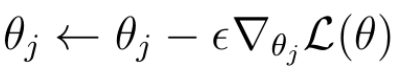Eq. 1 Gradient Descent for parameters θ with loss function L.

In this technique (Eq.1), we must calculate the gradient of the loss function L with respect to the weights (or parameters θ) that we want to improve. Subsequently, the weights/parameters are updated in the direction of the negative direction of the gradient.

By periodically applying the gradient descent to the weights, we will eventually arrive at the optimal weights that minimize the loss function and allow the neural network to make better predictions.

So far the theory.

Do not get me wrong, gradient descent is still a powerful technique. In practice, however, this technique may encounter certain problems during training that can slow down the learning process or, in the worst case, even prevent the algorithm from finding the optimal weights

These problems were on the one hand saddle points and local minima of the loss function, where the loss function becomes flat and the gradient goes to zero: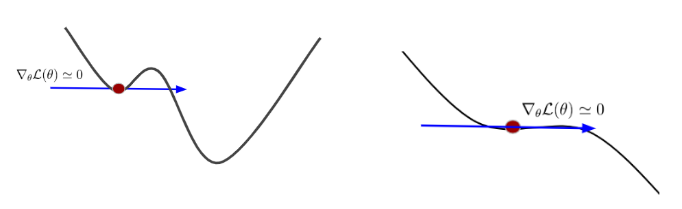Fig. 1 Saddle Points and Local Minima

A gradient near zero does not improve the weight parameters and prevents the entire learning process.

On the other hand, even if we have gradients that are not close to zero, the values of these gradients calculated for different data samples from the training set may vary in value and direction. We say that the gradients are noisy or have a lot of variances. This leads to a zigzag movement towards the optimal weights and can make learning much slower: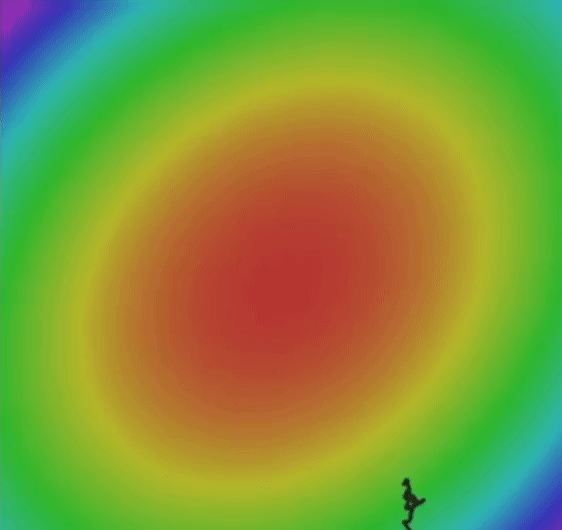Fig. 3 Example of zig-zag movements of noisy gradients.

In the following article, we are going to learn about more sophisticated gradient descent algorithms. All of these algorithms are based on the regular gradient descent optimization that we have come to know so far. But we can extend this regular approach for the weight optimization by some mathematical tricks to build even more effective optimization algorithms that allow our neural network to adequately handle these problems, thereby learning faster and to achieve a better performance

2. Stochastic Gradient Descent with Momentum

The first of the sophisticated algorithms I want to present you is called stochastic gradient descent with momentum.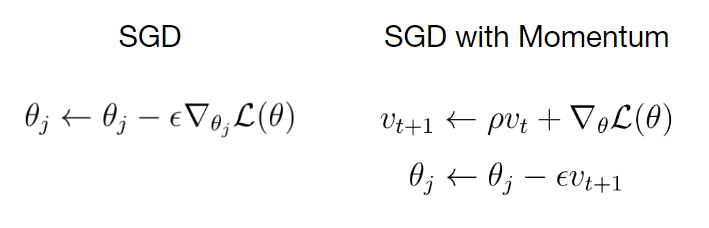Eq. 2 Equations for stochastic gradient descent with momentum.

On the left side in Eq. 2, you can see the equation for the weight updates according to the regular stochastic stochastic gradient descent. The equation on the right shows the rule for the weight updates according to the SGD with momentum. The momentum appears as an additional term ρ times v that is added to the regular update rule.

Intuitively speaking, by adding this momentum term we let our gradient to build up a kind of velocity v during training. The velocity is the running sum of gradients weighted by ρ.

ρ can be considered as friction that slows down the velocity a little bit. In general, you can see that the velocity builds up over time. By using the momentum term saddle points and local minima become less dangerous for the gradient. Because step sizes towards the global minimum now don’t depend only on the gradient of the loss function at the current point, but also on the velocity that has built up over time.

In other words, we are moving more towards the direction of velocity than towards the gradient at a certain point.

If you want to have a physical representation of the stochastic gradient descent with momentum think about a ball that rolls down a hill and builds up velocity over time. If this ball reaches some obstacles on its way, such as a hole or a flat ground with no downward slope, the velocity v would give the ball enough power to roll over these obstacles. In this case, the flat ground and the hole represent saddle points or local minima of a loss function.

In the following video (Fig. 4), I want to show you a direct comparison of regular stochastic gradient descent and stochastic gradient descent with momentum term. Both algorithms are trying to reach the global minimum of the loss function which lives in a 3D space. Please note how the momentum term makes the gradients to have less variance and fewer zig-zags movements.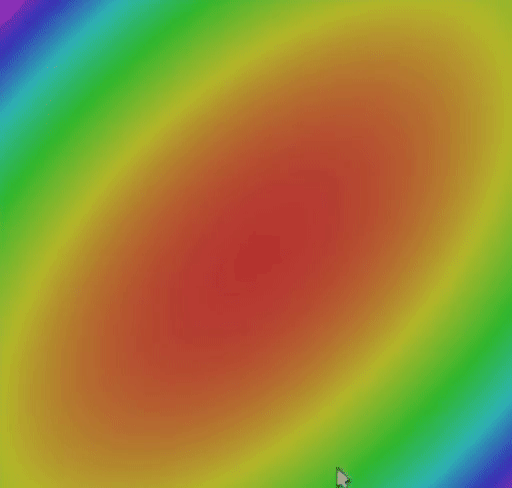Fig. 4 SGD vs. SGD with Momentum

In general, the momentum term makes converges towards optimal weights more stable and faster.

Another optimization strategy is called AdaGrad. The idea is that you keep the running sum of squared gradients during optimization. In this case, we have no momentum term, but an expression g that is the sum of the squared gradients.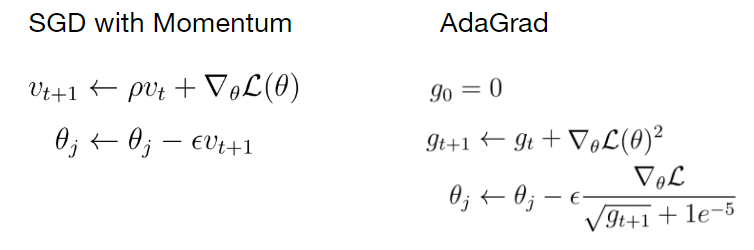Eq. 3 Parameter update rule for AdaGrad.

When we update a weight parameter, we divide the current gradient by the root of that term g. To explain the intuition behind AdaGrad, imagine a loss function in a two-dimensional space in which the gradient of the loss function in one direction is very small and very high in the other direction.

Summing up the gradients along the axis where the gradients are small causes the squared sum of these gradients to become even smaller. If during the update step, we divide the current gradient by a very small sum of squared gradients g, the result of that division becomes very high and vice versa for the other axis with high gradient values.

As a result, we force the algorithm to make updates in any direction with the same proportions.

This means that we accelerate the update process along the axis with small gradients by increasing the gradient along that axis. On the other hand, the updates along the axis with the large gradient slow down a bit.

However, there is a problem with this optimization algorithm. Imagine what would happen to the sum of the squared gradients when training takes a long time. Over time, this term would get bigger. If the current gradient is divided by this large number, the update step for the weights becomes very small. It is as if we were using very low learning that becomes even lower the longer the training goes. In the worst case, we would get stuck with AdaGrad and the training would go on forever.

4. RMSProp

There is a slight variation of AdaGrad called RMSProp that addresses the problem that AdaGrad has. With RMSProp we still keep the running sum of squared gradients but instead of letting that sum grow continuously over the period of training we let that sum actually decay.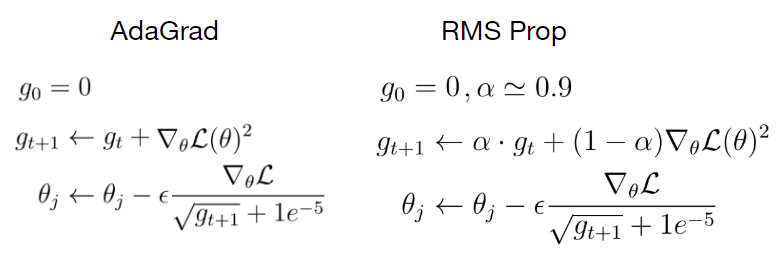Eq. 4 Update rule for RMS Prop.

In RMSProp we multiply the sum of squared gradients by a decay rate α and add the current gradient weighted by (1- α). The update step in the case of RMSProp looks exactly the same as in AdaGrad where we divide the current gradient by the sum of squared gradients to have this nice property of accelerating the movement along the one dimension and slowing down the movement along the other dimension.

Let’s see how RMSProp is doing in comparison with SGD and SGD with momentum in finding the optimal weights.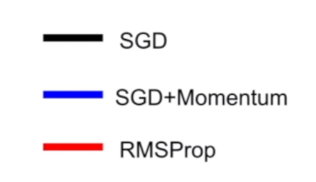Fig. 5 SGD vs. SGD with Momentum vs. RMS Prop

Although SGD with momentum is able to find the global minimum faster, this algorithm takes a much longer path, that could be dangerous. Because a longer path means more possible saddle points and local minima. RMSProp, on the other hand, goes straight towards the global minimum of the loss function without taking a detour.

So far we have used the moment term to build up the velocity of the gradient to update the weight parameter towards the direction of that velocity. In the case of AdaGrad and RMSProp, we used the sum of the squared gradients to scale the current gradient, so we could do weight updates with the same ratio in each dimension.

These two methods seemed pretty good ideas. Why do not we just take the best of both worlds and combine these ideas into a single algorithm?

This is the exact concept behind the final optimization algorithm called Adam, which I would like to introduce to you.

The main part of the algorithm consists of the following three equations. These equations may seem overwhelming at first, but if you look closely, you’ll see some familiarity with previous optimization algorithms.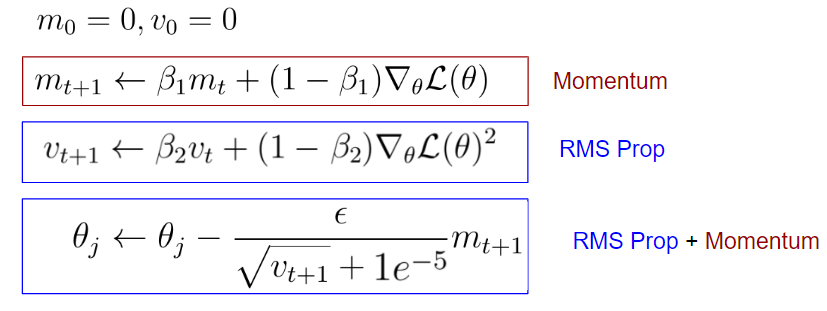Eq. 5 Parameter update rule for Adam Optimizer

The first equation looks a bit like the SGD with momentum. In the case, the term would be the velocity and the friction term. In the case of Adam, we call the first momentum and is just a hyperparameter.

The difference to SGD with momentum, however, is the factor (1- β1), which is multiplied with the current gradient.

The second part of the equations, on the other hand, can be regarded as RMSProp, in which we are keeping the running sum of squared gradients. Also, in this case, there is the factor (1-β2) which is multiplied with the squared gradient.

The term in the equation is called the second momentum and is also just a hyperparameter. The final update equation can be seen as a combination of RMSProp and SGD with Momentum.

So far, Adam has integrated the nice features of the two previous optimization algorithms, but here’s a little problem, and that’s the question of what happens in the beginning.

At the very first time step, the first and second momentum terms are initialized to zero. After the first update of the second momentum, this term is still very close to zero. When we update the weight parameters in the last equation, we divide by a very small second momentum term v, resulting in a very large first update step.

This first very large update step is not the result of the geometry of the problem, but it is an artifact of the fact that we have initialized the first and second momentum to zero. To solve the problems of large first update steps, Adam includes a correction clause: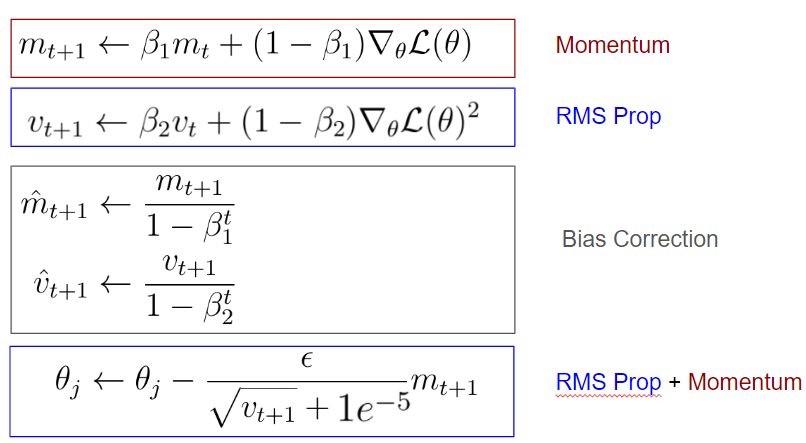Eq. 6 Bias Correction for Adam Optimizer

You can see that after the first update of the first and second momentum and we make an unbiased estimate of these momentums by taking into account the current time step. These correction terms make the values of the first and second momentum to be higher in the beginning than in the case without the bias correction.

As a result, the first update step of the neural network parameters does not get that large and we don’t mess up our training in the beginning. The additional bias corrections give us the full form of Adam Optimizer.

Now, let us compare all algorithms with each other in terms of finding the global minimum of the loss function: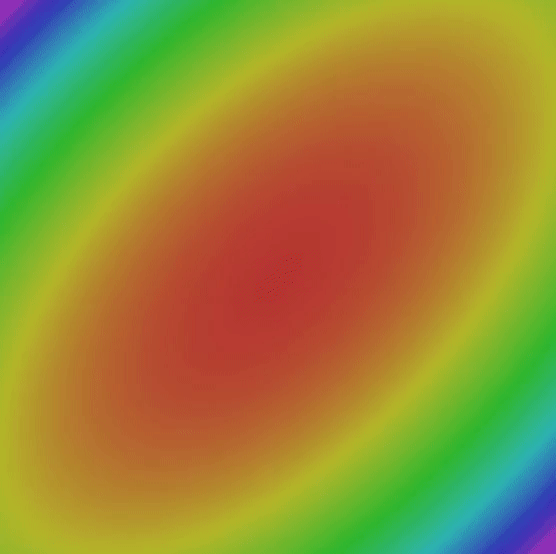Fig. 6 Comparison of all optimization algorithms.

6. What is the best Optimization Algorithm for Deep Learning?

Finally, we can discuss the question of what the best gradient descent algorithm is.

In general, a normal gradient descent algorithm is more than adequate for simpler tasks. If you are not satisfied with the accuracy of your model you can try out RMSprop or add a momentum term to your gradient descent algorithms.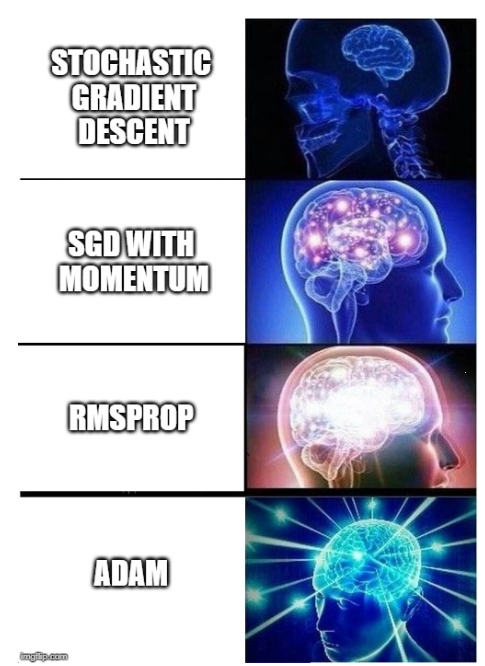But in my experience the best optimization algorithm for neural networks out there is Adam. This optimization algorithm works very well for almost any deep learning problem you will ever encounter. Especially if you set the hyperparameters to the following values:

• β1=0.9
• β2=0.999
• Learning rate = 0.001–0.0001

… this would be a very good starting point for any problem and virtually every type of neural network architecture I’ve ever worked with.

That’s why Adam Optimizer is my default optimization algorithm for every problem I want to solve. Only in very few cases do I switch to other optimization algorithms that I introduced earlier.

In this sense, I recommend that you always start with the Adam Optimizer, regardless of the architecture of the neural network of the problem domain you are dealing with.

How to build a Neural Network from scratch in PythonWhat’s a Neural Network?

Neural Networks are like the workhorses of Deep learning. With enough data and computational power, they can be used to solve most of the problems in deep learning. It is very easy to use a Python or R library to create a neural network and train it on any dataset and get a great accuracy.

Most introductory texts to Neural Networks brings up brain analogies when describing them. Without delving into brain analogies, I find it easier to simply describe Neural Networks as a mathematical function that maps a given input to a desired output.

Neural Networks consist of the following components

• An input layer, x
• An arbitrary amount of hidden layers
• An output layer, ŷ
• A set of weights and biases between each layer, W and b
• A choice of activation function for each hidden layer, σ. In this tutorial, we’ll use a Sigmoid activation function.

The diagram below shows the architecture of a 2-layer Neural Network (note that the input layer is typically excluded when counting the number of layers in a Neural Network)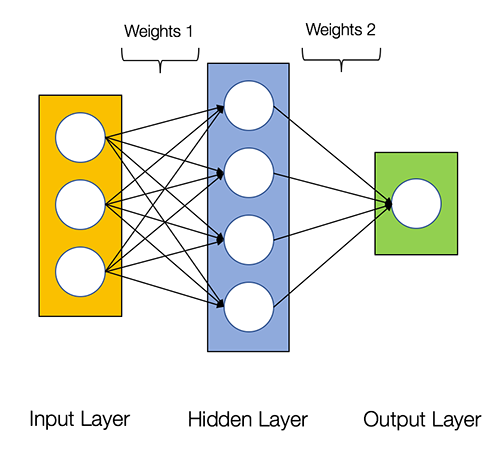<figcaption class="au ei od oe hr dn dl dm of og ap cu">Architecture of a 2-layer Neural Network</figcaption>

Creating a Neural Network class in Python is easy.

class NeuralNetwork:
def __init__(self, x, y):
self.input      = x
self.weights1   = np.random.rand(self.input.shape,4)
self.weights2   = np.random.rand(4,1)
self.y          = y
self.output     = np.zeros(y.shape)

Training the Neural Network

The output ŷ of a simple 2-layer Neural Network is: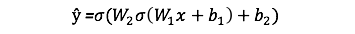You might notice that in the equation above, the weights W and the biases b are the only variables that affects the output ŷ.

Naturally, the right values for the weights and biases determines the strength of the predictions. The process of fine-tuning the weights and biases from the input data is known as training the Neural Network.

Each iteration of the training process consists of the following steps:

• Calculating the predicted output ŷ, known as feedforward
• Updating the weights and biases, known as backpropagation

The sequential graph below illustrates the process.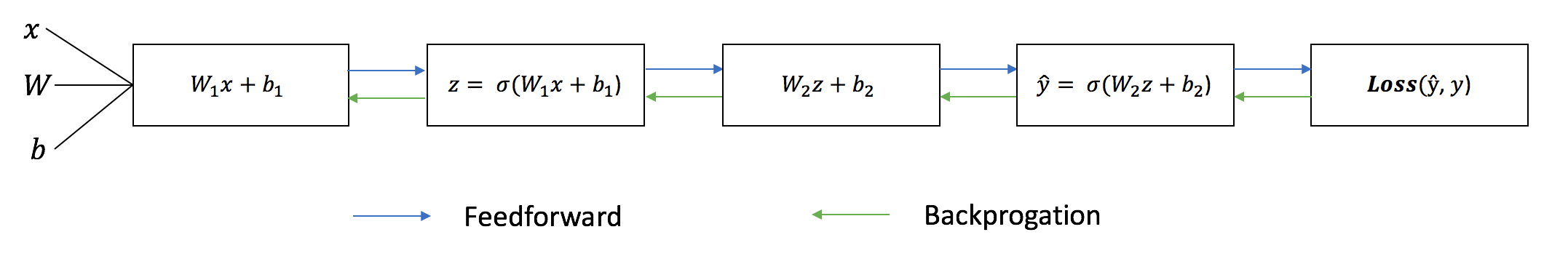Feedforward

As we’ve seen in the sequential graph above, feedforward is just simple calculus and for a basic 2-layer neural network, the output of the Neural Network is:Let’s add a feedforward function in our python code to do exactly that. Note that for simplicity, we have assumed the biases to be 0.

class NeuralNetwork:
def __init__(self, x, y):
self.input      = x
self.weights1   = np.random.rand(self.input.shape,4)
self.weights2   = np.random.rand(4,1)
self.y          = y
self.output     = np.zeros(self.y.shape)

def feedforward(self):
self.layer1 = sigmoid(np.dot(self.input, self.weights1))
self.output = sigmoid(np.dot(self.layer1, self.weights2))

However, we still need a way to evaluate the “goodness” of our predictions (i.e. how far off are our predictions)? The Loss Function allows us to do exactly that.

Loss Function

There are many available loss functions, and the nature of our problem should dictate our choice of loss function. In this tutorial, we’ll use a simple sum-of-sqaures error as our loss function.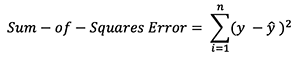That is, the sum-of-squares error is simply the sum of the difference between each predicted value and the actual value. The difference is squared so that we measure the absolute value of the difference.

Our goal in training is to find the best set of weights and biases that minimizes the loss function.

Backpropagation

Now that we’ve measured the error of our prediction (loss), we need to find a way to propagate the error back, and to update our weights and biases.

In order to know the appropriate amount to adjust the weights and biases by, we need to know the derivative of the loss function with respect to the weights and biases.

Recall from calculus that the derivative of a function is simply the slope of the function.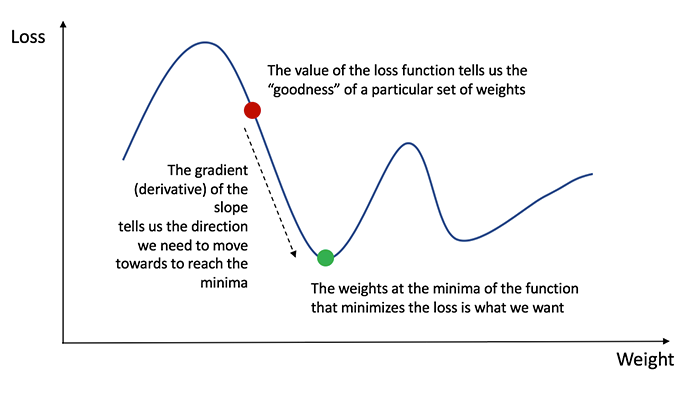<figcaption class="au ei od oe hr dn dl dm of og ap cu">Gradient descent algorithm</figcaption>

If we have the derivative, we can simply update the weights and biases by increasing/reducing with it(refer to the diagram above). This is known as gradient descent.

However, we can’t directly calculate the derivative of the loss function with respect to the weights and biases because the equation of the loss function does not contain the weights and biases. Therefore, we need the chain rule to help us calculate it.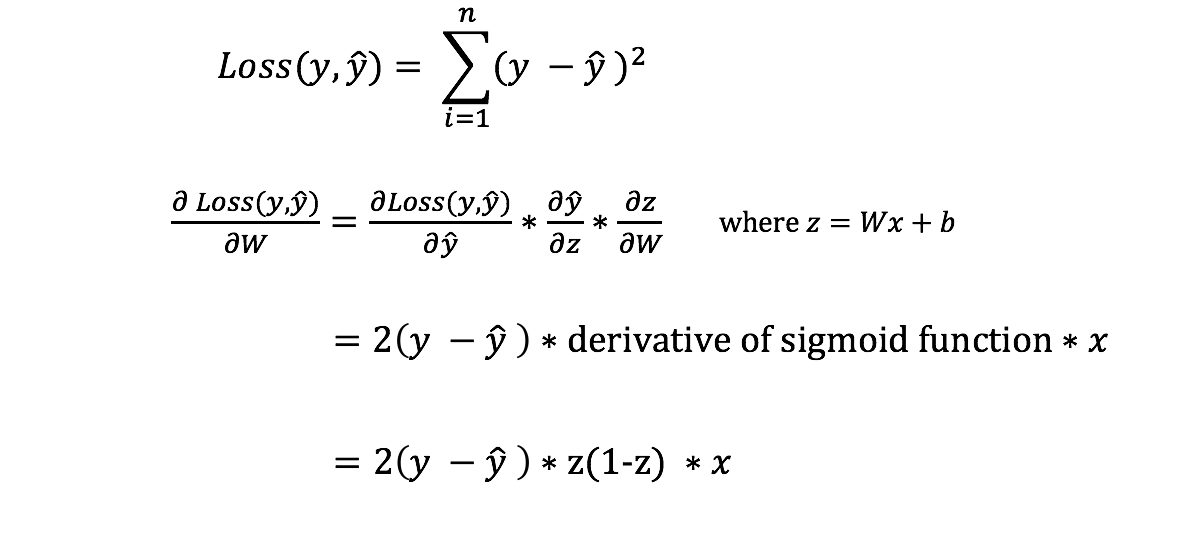<figcaption class="au ei od oe hr dn dl dm of og ap cu">Chain rule for calculating derivative of the loss function with respect to the weights. Note that for simplicity, we have only displayed the partial derivative assuming a 1-layer Neural Network.</figcaption>

Phew! That was ugly but it allows us to get what we needed — the derivative (slope) of the loss function with respect to the weights, so that we can adjust the weights accordingly.

Now that we have that, let’s add the backpropagation function into our python code.

class NeuralNetwork:
def __init__(self, x, y):
self.input      = x
self.weights1   = np.random.rand(self.input.shape,4)
self.weights2   = np.random.rand(4,1)
self.y          = y
self.output     = np.zeros(self.y.shape)

def feedforward(self):
self.layer1 = sigmoid(np.dot(self.input, self.weights1))
self.output = sigmoid(np.dot(self.layer1, self.weights2))

def backprop(self):
# application of the chain rule to find derivative of the loss function with respect to weights2 and weights1
d_weights2 = np.dot(self.layer1.T, (2*(self.y - self.output) * sigmoid_derivative(self.output)))
d_weights1 = np.dot(self.input.T,  (np.dot(2*(self.y - self.output) * sigmoid_derivative(self.output), self.weights2.T) * sigmoid_derivative(self.layer1)))

# update the weights with the derivative (slope) of the loss function
self.weights1 += d_weights1
self.weights2 += d_weights2

For a deeper understanding of the application of calculus and the chain rule in backpropagation, I strongly recommend this tutorial by 3Blue1Brown.

<iframe width="560" height="315" src="https://www.youtube.com/embed/tIeHLnjs5U8" frameborder="0" allow="accelerometer; autoplay; encrypted-media; gyroscope; picture-in-picture" allowfullscreen></iframe>

Putting it all together

Now that we have our complete python code for doing feedforward and backpropagation, let’s apply our Neural Network on an example and see how well it does.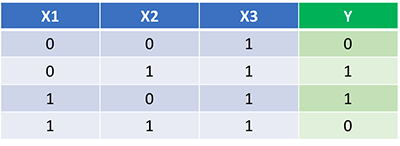Our Neural Network should learn the ideal set of weights to represent this function. Note that it isn’t exactly trivial for us to work out the weights just by inspection alone.

Let’s train the Neural Network for 1500 iterations and see what happens. Looking at the loss per iteration graph below, we can clearly see the loss monotonically decreasing towards a minimum. This is consistent with the gradient descent algorithm that we’ve discussed earlier.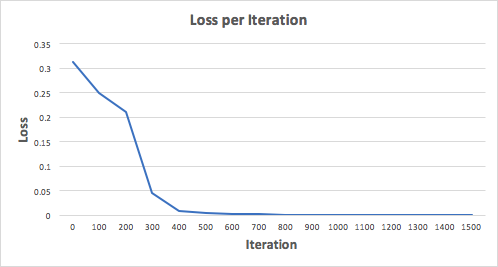Let’s look at the final prediction (output) from the Neural Network after 1500 iterations.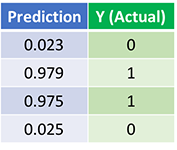<figcaption class="au ei od oe hr dn dl dm of og ap cu">Predictions after 1500 training iterations</figcaption>

We did it! Our feedforward and backpropagation algorithm trained the Neural Network successfully and the predictions converged on the true values.

Note that there’s a slight difference between the predictions and the actual values. This is desirable, as it prevents overfitting and allows the Neural Network to generalize better to unseen data.

What’s Next?

Fortunately for us, our journey isn’t over. There’s still much to learn about Neural Networks and Deep Learning. For example:

• What other activation function can we use besides the Sigmoid function?
• Using a learning rate when training the Neural Network
• Using convolutions for image classification tasks

I’ll be writing more on these topics soon, so do follow me on Medium and keep and eye out for them!

Final Thoughts

I’ve certainly learnt a lot writing my own Neural Network from scratch.

Although Deep Learning libraries such as TensorFlow and Keras makes it easy to build deep nets without fully understanding the inner workings of a Neural Network, I find that it’s beneficial for aspiring data scientist to gain a deeper understanding of Neural Networks.

This exercise has been a great investment of my time, and I hope that it’ll be useful for you as well!

Tensorflow: Logits and labels must have the same first dimension

I'm new to machine learning in TF. I have this dataset which I generated and exported into a .csv file. It is here: tftest.csv.

The 'distributions' column corresponds to a unique system of equations which I have tried to condense down into a series of digits in SageMath. The 'probs' column correspond to whether one should mutiply a given equation by a given monomial of the equation, based on the row and column it is located in. The above is just for overview and is not related to my actual question.

Anyways, here's my code. I've tried to explain it as best as I can with annotations.

<pre class="ql-syntax" spellcheck="false">import csv import numpy as np import matplotlib.pyplot as plt import tensorflow as tf import tensorflow.keras as keras

distribution_train = []
probs_train = []

y_train = []

with open('tftest.csv') as csv_file:

for row in csv_reader:
distribution_train.append(row)
probs_train.append(row)

'''
Get rid of the titles in the csv file
'''
distribution_train.pop(0)
probs_train.pop(0)

'''
For some reason everything in my csv file is stored as strings.
The below function is to convert it into floats so that TF can work with it.
'''
def num_converter_flatten(csv_list):
f = []
for j in range(len(csv_list)):
append_this = []
for i in csv_list[j]:
if i == '1' or i == '2' or i == '3' or i == '4' or i == '5' or i == '6' or i == '7' or i == '8' or i =='9' or i =='0':
append_this.append(float(i))
f.append((append_this))

return f

x_train = num_converter_flatten(distribution_train)
y_train = num_converter_flatten(probs_train)

x_train = tf.keras.utils.normalize(x_train, axis=1)
y_train = tf.keras.utils.normalize(y_train, axis=1)

model = tf.keras.models.Sequential()

'''
I'm making the final layer 80 because I want TF to output the size of the
'probs' list in the csv file
'''